# How to calculate the bond price in Excel?

In this tutorial, we will demonstrate how you can calculate the price of a zero coupon bond, the price of an annual coupon bond, and the price of the semi-annual coupon bond in an Excel sheet.

## Calculating the Price of a Zero Coupon Bond in Excel

Consider an example, there is a 10 years bond and the value is 5000, and the rate of interest is 7.5 %. Before the maturity date, the bondholder cannot get any coupon. You can calculate the price of the zero bonds using the formula.

### Step 1

Open an Excel sheet and enter the data as shown in the below screenshot for your reference.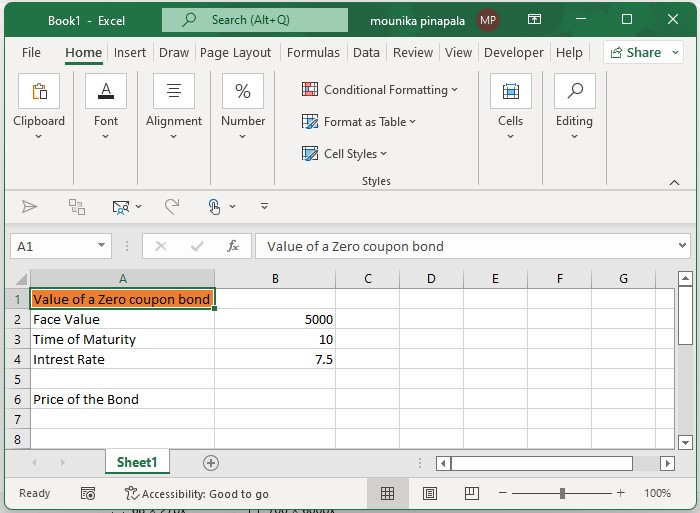### Step 2

Now you need to enter the below-given formula and press the enter key as shown in the below screenshot for your reference.

=PV(B4,B3,0,B2)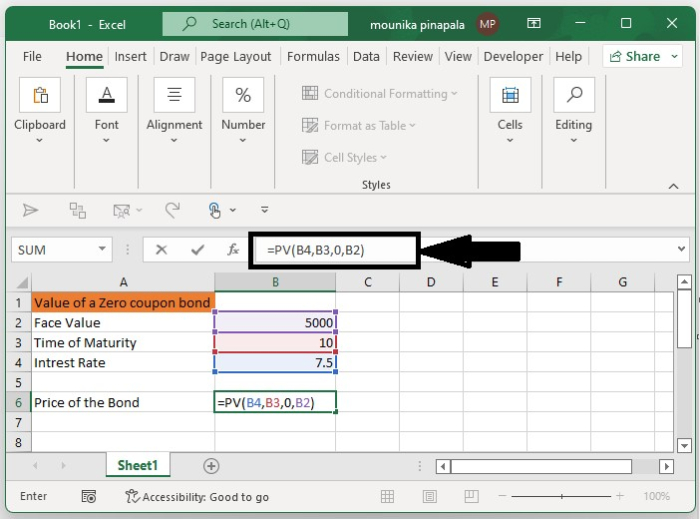Note − In the above-given formula, B4 – is the rate of interest, B3 – is the maturity year, 0 – means no coupon, and B2 – is the face value, you can change all this as per your need.

## Calculating the Annual Coupon Bond in Excel

Consider there is an annual coupon bond where bondholder can get the coupon every year. You can calculate the price of the annual coupon bond using below.

### Step 1

Open an excel sheet and enter the data as shown in the below screenshot for your reference.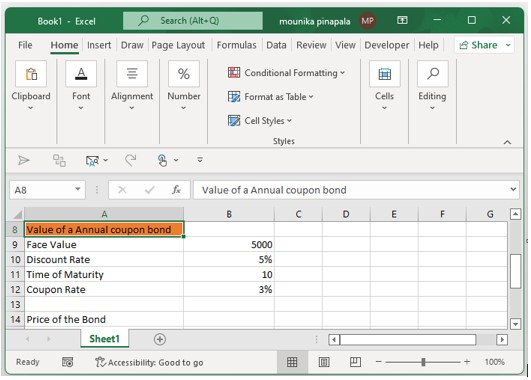### Step 2

Now you need to enter the below given formula and press the enter key as shown in the below screenshot for your reference.

=PV(B10,B11,(B9*B12),B9)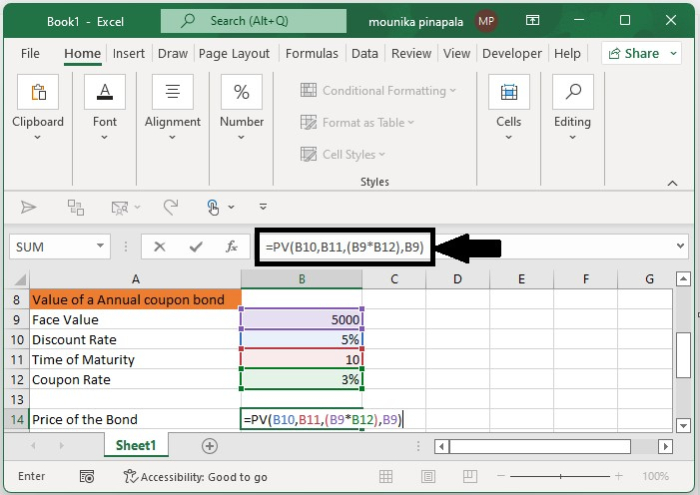Note − In the above formula, B10 is the interest rate, B11 is the maturity year, B9 is the face value, and B9*B12 is the coupon you will get every year. You can change this as per your need.

## Calculating the Semi-Annual Coupon Bond in Excel

At times bondholders can get coupons twice a year. To calculate the bond price in this scenario, follow the steps given below −

### Step 1

Open an excel sheet and enter the data as shown in the below screenshot for your reference.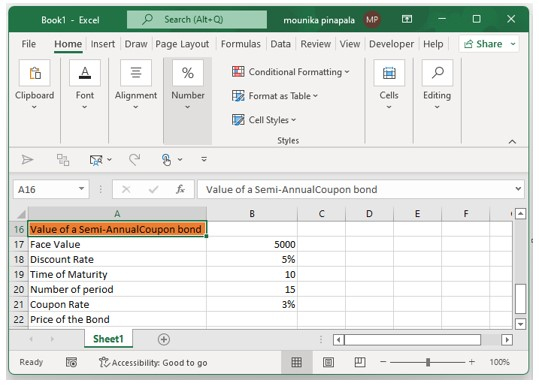### Step 2

Now you need to enter the below given formula and press the enter key as shown in the below screenshot for your reference.

=PV(B18/2,B20,B17*B20/2,B17)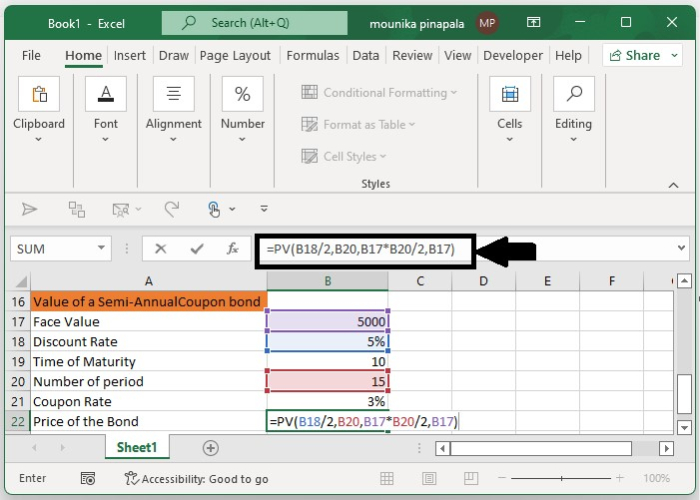Note − In the above formula, B18 is the annual rate of interest, B20 is the actual period, B17*B21 gets the coupon, and B17 is the face value. You can change this as per your need.

## Conclusion

In this tutorial, we used a set of simple examples to show how you can calculate the price of different types of coupon bonds in an Excel sheet.

Updated on: 06-Feb-2023

606 Views